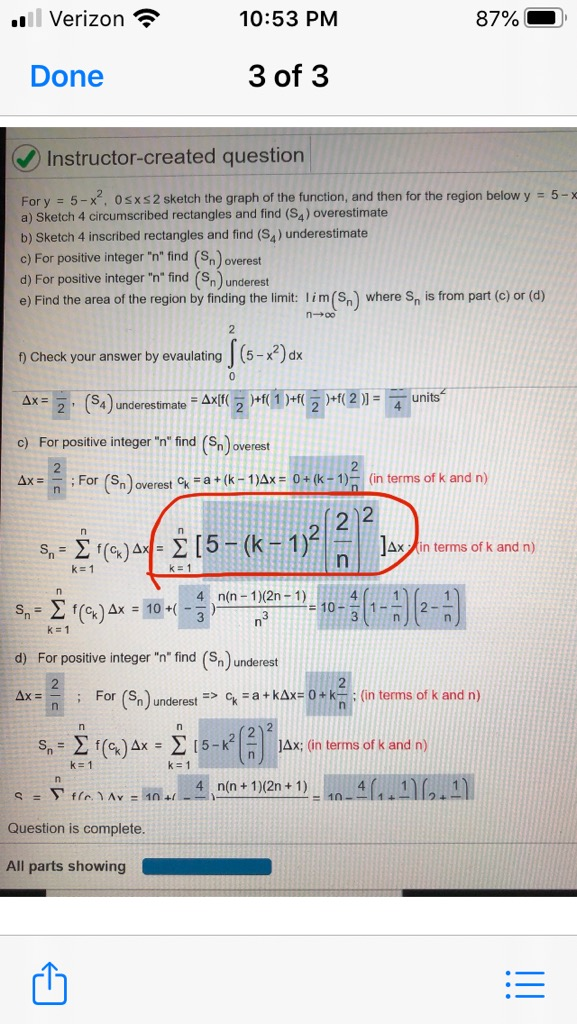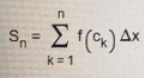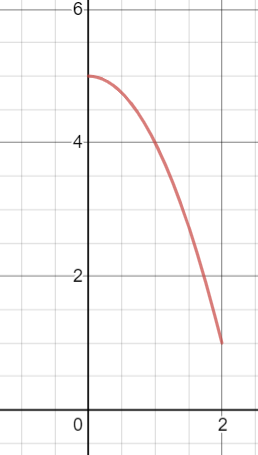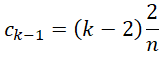# l Verizon ?10:53 PM87%Done3 of 3Instructor-created questionFor y = 5-x, Osxs2 sketch the graph of the function, and then for the region below y = 5-xa) Sketch 4 circumscribed rectangles and find (Sa) overestimateb) Sketch 4 inscribed rectangles and find (S,) underestimatec) For positive integer "n" find (Sn) overestd) For positive integer "n" find (Sn) undereste) Find the area of the region by finding the limit: lim(S,) where S, is from part (c) or (d)|(5-x²) dxf) Check your answer by evaulating)+f( 1 )+f()+f( 2 )] =unitsAx =2: S4) underestimate = Ax[f(5c) For positive integer "n" find (Sn) overestAx =; For (S)overest k =a+ (k -1)Ax = 0+ (k - 1)- (in terms of k and n)2 2JAx in terms of k and n)S,Σ) ΔΣ15-(Κ- 1ink= 1пS, = x)Ax = 10 +( -34 n(n - 1)(2n - 1)4.=10-2-n°k=1d) For positive integer "n" find (Sn) underest2Ax =; For (S)underest => C = a+kAx= 0 + k-: (in terms of k and n)s,- Σ ) Δκ Σ15-κJAx; (in terms of k and n)k= 1k=1n(n + 1)(2n + 1)S = Y flcAy = 10 +10-Question is complete.All parts showing!!

Question
1 viewshelp_outlineImage Transcriptionclosel Verizon ? 10:53 PM 87% Done 3 of 3 Instructor-created question For y = 5-x, Osxs2 sketch the graph of the function, and then for the region below y = 5-x a) Sketch 4 circumscribed rectangles and find (Sa) overestimate b) Sketch 4 inscribed rectangles and find (S,) underestimate c) For positive integer "n" find (Sn) overest d) For positive integer "n" find (Sn) underest e) Find the area of the region by finding the limit: lim(S,) where S, is from part (c) or (d) |(5-x²) dx f) Check your answer by evaulating )+f( 1 )+f( )+f( 2 )] = units Ax = 2: S4) underestimate = Ax[f(5 c) For positive integer "n" find (Sn) overest Ax = ; For (S)overest k =a+ (k -1)Ax = 0+ (k - 1)- (in terms of k and n) 2 2 JAx in terms of k and n) S,Σ) ΔΣ15-(Κ- 1 in k= 1 п S, = x) Ax = 10 +( - 3 4 n(n - 1)(2n - 1) 4. =10- 2- n° k=1 d) For positive integer "n" find (Sn) underest 2 Ax = ; For (S)underest => C = a+kAx= 0 + k-: (in terms of k and n) s,- Σ ) Δκ Σ15-κ JAx; (in terms of k and n) k= 1 k=1 n(n + 1)(2n + 1) S = Y flcAy = 10 + 10- Question is complete. All parts showing !! fullscreen
check_circle

Step 1

Hi! We are answering for the red circulated part of the question.

Note:

For over estimation (upper Riemann sum),where, f(ck) = sup f([ck-1, ck]).

Step 2

Let us draw the graph for better understanding.Notice that the function is a strictly decreasing function in the interval [0,2].

So, the supremum of the function will exists at ck-1 in the interval [ck-1, ck].

And...

### Want to see the full answer?

See Solution

#### Want to see this answer and more?

Solutions are written by subject experts who are available 24/7. Questions are typically answered within 1 hour.*

See Solution
*Response times may vary by subject and question.
Tagged in